# Department of Mathematics

## Areas of Research

At the DASIV Center, we are passionate about research. Our faculty members have earned international recognition for their research excellence. On this page, you can find the latest updates on their ongoing projects.

### Current Projects

• Regularity and Singularity Formation in Swarming and Related Fluid Models
• State and Parameter Estimation for High Dimensional Parametric Partial Differential Models
• Data Assimilation
• Non-linear Model Reduction
• Stable Variational Formulations for Transport Dominated, Especially based on Discontinuous Petrov Galerkin Concepts, and Non-linear Problems
• Hyper Spectral Imaging, in Particular EDX Tomography

### Focus Points

Driving aspects in research on large scale numerical simulation based on partial differential equation models are (i) the ability of efficiently solve discrete problems of unprecendented size, typically stemming from discretizations of underlying continuous models, and (ii) assessing the accuracy of the numerical result with regard to the actual continuous quantity of interest.

These two issues are traditionally treated (almost) separately following the traditional approach "first discretize then analyze". This is oversimplified to stress the following point. Of course, resorting to expert knowledge and a priori information one contrives a discretization, tries to understand the discrete problem and explores possibly efficient ways of solving it, often with as little reference to the underlying continuous problem as possible. Then in step (ii), tapping into background theory, most notably regularity theory or approximation theory, the actual accuracy of the numerical output with regard to the continuous solution is assessed. When resorting to a priori estimates one often ignores somewhat that some of the involved quantities may not be known or quantifiable or that some of the assumptions may actually not hold. Nevertheless, this approach has shown great success for the class of linear (or even semi- and some quasi-linear) elliptic problems where both mathematical and numerical analysis have by now been well developed.

This is by far less the case for other relevant problem classes such as indefinite, singularly perturbed, transport dominated, or many nonlinear problem types and problems involving both local and nonlocal operators. Then often the enormous challenges encountered already in (i) absorb almost all attention while (ii) gets palpably replaced by less rigorous validation means such as comparison with experiments or even visual inspection. This has severe adverse implications when addressing Uncertainty Quantification (UQ) tasks related to such poblems. In fact, how would one seriously quantify the propagation of uncertain data through a given model if the discretization error by itself cannot be quantified.

A central theme in our research related to PDE based models is to develop concepts for a quantifiable and certified accuracy assessment also for problem classes far beyond the realm of elliptic problems. Roughly speaking a guiding thread is to reverse the above order "first discretize then analyze" by "sticking to the continuous problem as long as possible" so as to best exploit intrinsic model structures at all computational stages. A key role is played by identifying first in a suitable infinite dimensional function space setting an idela iteration that needs to be shown to converge to the continuous solution with a fixed error reduction per spep. Ideally each iteration involves a linear problem for which a stable variational formulation has to be identified. The employed stability notion entails a tight error-residual relationship which provides the foundation for deriving tight a posteriori error bounds. The by now well-developed adaptive solution concepts for elliptic problems can be viewed as special instances of this more general framework, see also the current work in "hp" project. The common ground for all these work is the availability of stable symmetric variational formulations, coercivity or monotonicity. Transport dominated problems are examples where the use of unsymmetric variational formulations is mandatory for stability on both the infinite and finite dimensional level. For problem scenarios of current interest this is often no longer the case. An important numerical work horse is then Discontinuous Petrov Galerkin (DPG) methodology which conveniently allows one to tightly interrelate the infinite dimensional and discrete problem. We have recently been studying such methods in the context of transport dominated problems. A Sample Project: The latter ones play an important role in contriving an error controlled solver for radiative transfer. Specifically, this treats the simple model problem

$$(\mathcal{T}_\vec{s} -\cal K_\vec{s})u :=\vec{s}\cdot\nabla u(x,\vec{s}) + \sigma(x,\vec{s}) u(x,\vec{s}) - \int_\S K(x,\vec{s}',\vec{s})u(x,\vec{s}')\,d\vec{s}' = f(x,\vec{s})$$

complemented by suitable "inflow-boundary conditions". Such kinetik models describe the propagation of particles in a collisional medium modeling, e.g., heat transfer phenomena, neutron transport or medical imaging processes. Evident obstructions are the presence of the global integral operator modeling scattering, the lack of stabilizing dffusive terms in the differential operator, the fact that solutions not only depend on spatial but also on velocity variables, and the typically very low regularity of solutions which is not properly measured in classical Sobolev scales. Based on identifying first suitable Petrov Galerkin type stable variational formulations, idela iterations of the form

$$u_{n+1}=\mathcal{T}^{-1}(\cal K u_n + f),\quad n\in \mathbb{N}_0,$$

are shown to converge to the exact solution with a fixed error reduction per step. The numerical method realizes this iteration approximately within suitable dynamically updated error tolerances. The approximate inversion of the pure transport operator T relies on an error controlled solution of the involved "fiber transport problems" with respect to adaptively selected transport directiosn, see Figure 0.1.

Figure 0.1: Adaptive meshes for fiber transport solutions with respect to two different directions and merged mesh (right)

••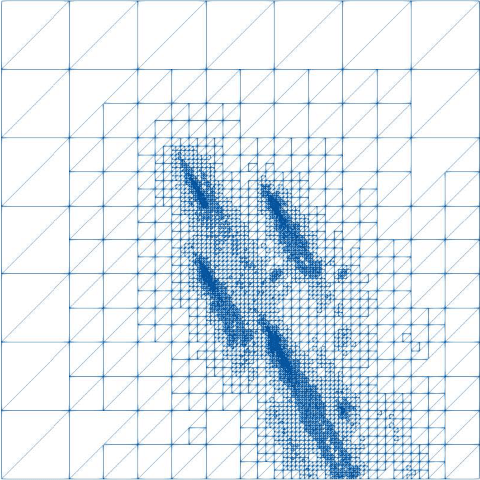•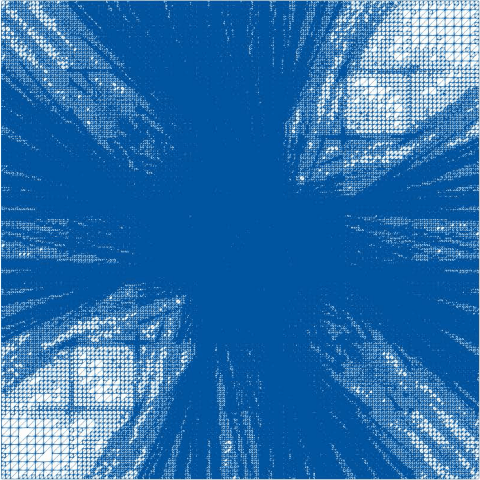In this example the source term and the absorbtion coefficient are rough, namely piecewise constants with a checkerboard pattern. This is reflected by the "expected" solution obtained by integrating over the direction field.

Figure 0.2: left: Checkerboard structure of absorbtion coefficient σ and source term f; middle: convergence history for up to 10 outer iteration steps. The light and dark blue curves show the achieved accuracy levels for the approximate transport solves and approximate aplications of the scatterer, called at the respective outer iteration step. The purple curve reflects the overall accuracy; right: integrated over S ("expected") solutions after 10 iterations.

••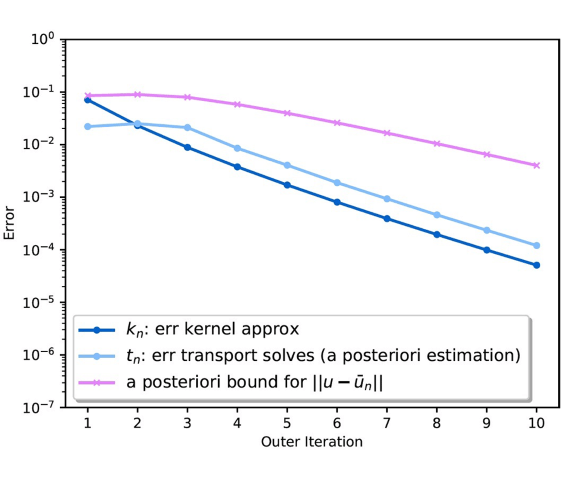•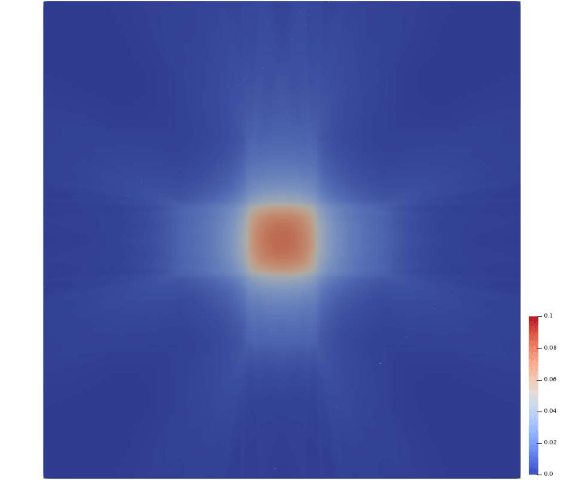Finite element methods approximate solutions to partial differential equations.

The approximation models the geometry of the region by a grid of elements. To improve a given approximation, smaller elements must be used, so as to be able to capture finer details. Of course, if the elements get smaller, more of them must be used, and this raises the cost of a more accurate approximation. An adaptive finite element method gauges the regions where it believes the current approximation is poor, and only shrinks elements in those areas, thus controlling the cost.

The Discontinuous Petrov Galerkin method can provide a set of error indicators that guide such an adaptive algorithm. Current ongoing research on difficult problems such as the p-Laplacian uses these error indicators to intelligently adjust the finite element mesh.

For a simple illustration of how this process evolves, consider the sequence of meshes generated in a model computation on the unit square.

•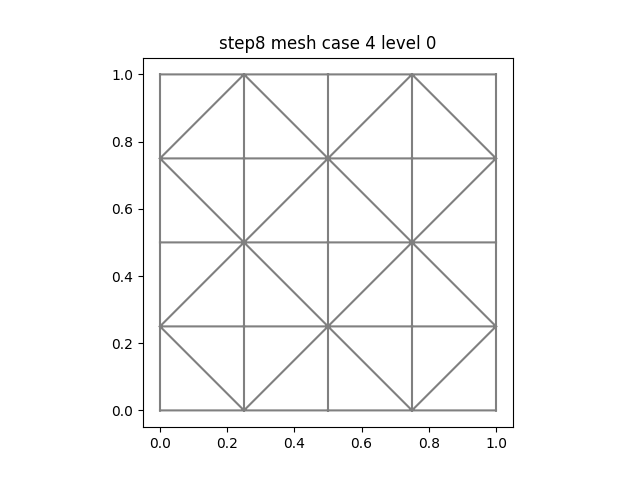••The refinement of the mesh corresponds to regions where the solution itself is changing rapidly:

•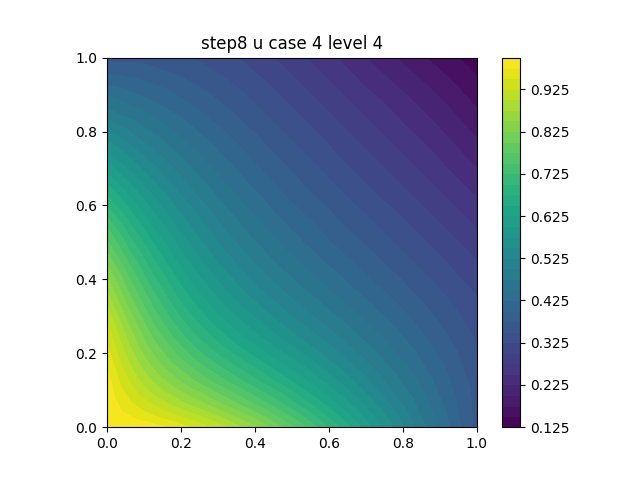Good adaptive meshing is critical in cases where the solution has sharp variations, where the boundary conditions or coefficient functions have jumps or steep gradients, or where the geometry itself offers difficulties because of indentations, obstructions, or variations in scale.

Mathematical/physical models are never exact. On the other hand, to be able to faithfully predict complex processes merely based on measurements or observational data, would require more data than typically available. Even an approximate model for an observed process offers therefore indispensible priors for accurately interpreting data. Primarily in the framework of parametric PDE models part of our present research addresses fundamental as- pects of Uncertainty Quantification for forward simulations as well as inverse tasks such as state and parameter estimation. In [?] one finds a general algorithmic template that can be specified to sparse polynomial expansion schemes, to low-rank approximations splitting spatial and parametric variables, as well as fully separable hierarchical tensor approximations for high dimensional parametric PDE solutions. These algorithms are fully adaptive and a posteriori error controlled. They are schown to exhibit near-optimal convergence and complexity for relevant benchmark classes. [?] approaches data assimilation in a broad sense as an optimal recovery problem and identifies intrinsic estimation limits along with optimal algorithms. Im important natural role in forward simulations as well as inverse problems in this context is played by certifiable reduced bases.

Imaging with electrons and other particles, in particular using scanning transmission electron microscopy (STEM), will become increasingly important in the near future, especially in materials and life sciences . While optical microscopes could perform close to their physical limit of about 200 nm, STEM encounters practical limits before the fundamental limit is reached. Due to the serial acquisition of the image, instabilities in the position of the probe introduce random and systematic errors in the positions of the atomic columns. Our goal is to lower these practical limits using better mathematical models to alleviate these errors. In collaboration with the Nano Center at USC led by Prof. T. Vogt, the group of Prof. P. Voyles at the University of Wisconsin, Madison, and Prof. B. Berkels, RWTH Aachen, Germany, we were able to lower the practical limits achieving unprecedented precision below 1 pm using our non-rigid registration approach  and . The pictures below are from ; the first shows the original first frame of a series of 512 images and the second shows the result of their averaging using our method. The precision in measuring the distance between the red dots is below 1 pm, which is 5 to 7 times better than the previous results.

•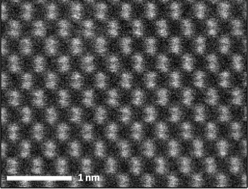First frame

•Result of averaging

Collective behaviors are widely manifested in many biological contexts: schools of fish, flocks of birds, colonies of ants, etc. Such complex self-organized systems have attracted a lot of attention to scientists, including mathematicians. Over the last decade, a lot of effort has been made to develop a mathematical framework to understand global behaviors in complex biological and social systems.

In particular, our research focus on models of self-organized dynamics, investigating the emergence of small-scale interactions towards global phenomena, such as flocking, swarming and clustering. A multi-scale framework originally developed for gas dynamics can be adapted to these biological systems, to efficiently reduce the size of the large systems. Powerful tools in kinetic theory and fluid mechanics are being applied and further developed to understand the nonlinear and nonlocal structures of the systems, as well as the connections to real-world applications.

Numerical implementations are also being investigated for the complex kinetic systems, featuring structural preserving, and dimension reduction.